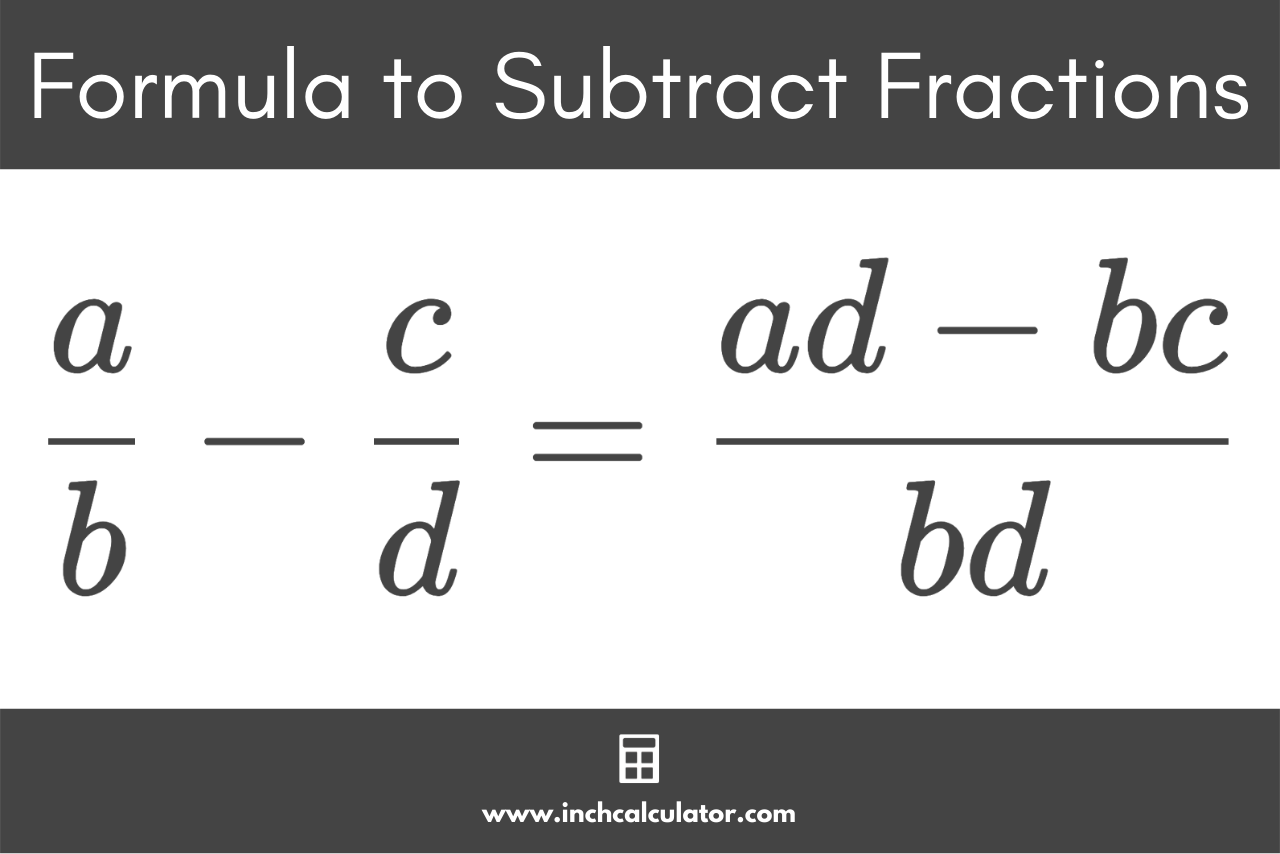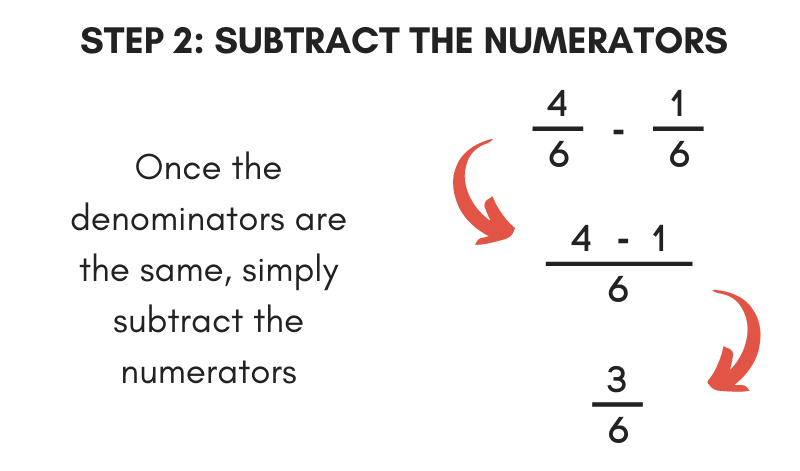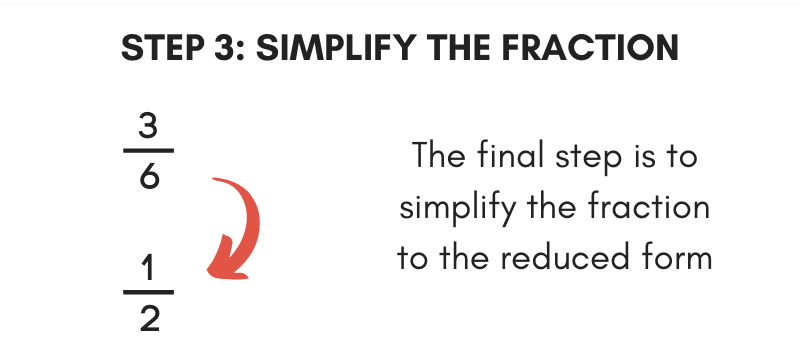# Subtracting Fractions Calculator

Subtract one fraction from another by entering them below. You can optionally add a whole number to subtract a mixed fraction as well.

Fraction One:
-
Fraction Two:

## Result as a Fraction:

2 / 3-1 / 6=1 / 2

## Result as a Decimal:

2 / 3-1 / 6=0.5

### Steps to Solve Expression with Fractions

Find the least common denominator so that all of the denominators match.
The least common denominator of 3 and 6 is 6
Find the multiple for each fraction that can be multiplied by the denominator to get the least common denominator.
Find the multiple by dividing the least common denominator by each fraction's denominator.
Fraction 1 Multiple: 6 ÷ 3 = 2
Fraction 2 Multiple: 6 ÷ 6 = 1
Multiply each fraction's numerator and denominator by the multiple.
2 × 2 / 3 × 2-1 × 1 / 6 × 1=4 / 6-1 / 6
Now that the denominators of each fraction are the same, subtract the second numerator from the first.
4 - 1 / 6=3 / 6
Reduce the fraction by finding the greatest common factor (GCF) of the numerator and denominator.
GCF of 3 and 6 = 3
Divide the numerator and denominator by the greatest common factor (3).
3 ÷ 3 / 6 ÷ 3=1 / 2
Find the decimal by dividing the numerator by the denominator.
1 / 2=1÷2=0.5
Learn how we calculated this below

## How to Subtract Fractions

A fraction is a numerical value that is not a whole number, and it generally represents a portion of a whole (e.g. one slice of a pie).

Subtracting fractions is a bit different from subtracting regular whole numbers, but you can use a few methods to do it.

### Fraction Subtraction Formula

You can use the following formula to subtract fractions.

a / bc / d = ad – bc / bd

To subtract two fractions, you can multiply the numerator of the first fraction with the denominator of the second fraction. Then, subtract it from the product of the numerator of the second fraction with the denominator of the first fraction, and write the result over the bottom of both fractions.By multiplying the denominators together, The formula ensures that both denominators match and are both raised to a common denominator by multiplying them together.

Since the numerators are also multiplied using the same multiple as the denominator, the result is an equivalent fraction.

Then, the resulting numerators can be subtracted using normal means.

You can put this formula to practice and subtract fractions in three easy steps.

### Step One: Convert to Fractions with a Common DenominatorThe first step to subtracting fractions is to make the denominators of each fraction match. Convert each one to an equivalent fraction with a matching denominator to the other.

#### Find the Lowest Common Denominator

You’ll need to find the lowest common denominator for each of the fraction’s denominators. The lowest common denominator is the smallest number that each denominator can evenly divide into.

#### Find the Multiplier

Next, find the multiple for each denominator that can be multiplied to equal the lowest common denominator. To find the multiple for each fraction, divide the least common denominator by the denominator.

#### Multiply by the Multiplier

Then, multiply both the top and bottom number of the fraction by the multiple to find the equivalent fractions with matching denominators. You can also use an equivalent fractions calculator to find one with a matching denominator.

For example, let’s convert the fractions 1 / 2 and 1 / 3 to fractions with the same denominator.

The least common denominator of 2 and 3 is 6.

Find the multiple for 1 / 2
multiple = lcd ÷ denominator
multiple = 6 ÷ 2 = 3

Find the equivalent fraction of 1 / 2 using the multiple 3
1 / 2 = 1 × 3 / 2 × 3
1 / 2 = 3 / 6

Find the multiple for 1 / 4
multiple = lcd ÷ denominator
multiple = 6 ÷ 3 = 2

Find the equivalent fraction of 1 / 3using the multiple 2
1 / 3 = 1 × 2 / 3 × 2
1 / 3 = 2 / 6

Thus, the equivalent fractions of 1 / 2 and 1 / 3 are 3 / 6 and 2 / 6

### Step Two: Subtract the NumeratorsOnce the bottom numbers of each fraction match, subtract the top numbers from each together to find the result.

Simply subtract the second numerator from the first, then put the result over the common denominator.

For example, let’s continue the previous example and subtract 2 / 6 from 3 / 6.

3 / 62 / 6 = 3-2 / 6
3 / 62 / 6 = 1 / 6

### Step Three: Simplify the FractionThe final step to subtracting fractions is to reduce the result to its simplest form. Start by finding the greatest common factor of the top and bottom parts of the result.

Then, divide both the top and bottom numbers by the greatest common factor to reduce it. An even easier way to reduce is to use our fraction simplifier.

You can also subtract fractions using our fraction calculator.